# Introduction to PyMC¶

While Markov chain Monte Carlo is a powerful method for fitting Bayesian models, they can be difficult to apply generally, as most commercial statistical analysis packages do not implement it. This has been an impediment to the growth of Bayesian methods. PyMC is a python module that implements Bayesian statistical models and fitting algorithms, including MCMC. Its flexibility and extensibility make it applicable to a large suite of problems. Along with core sampling functionality, PyMC includes methods for summarizing output, plotting, goodness-of-fit and convergence diagnostics.

PyMC 2.3 provides functionalities to make Bayesian analysis as painless as possible. Here is a short list of some of its features:

• Fits Bayesian statistical models with Markov chain Monte Carlo and other algorithms.
• Includes a large suite of well-documented statistical distributions.
• Uses NumPy for numerics wherever possible.
• Includes a module for modeling Gaussian processes.
• Sampling loops can be paused and tuned manually, or saved and restarted later.
• Creates summaries including tables and plots.
• Traces can be saved to the disk as plain text, Python pickles, SQLite or MySQL database, or hdf5 archives.
• Several convergence diagnostics are available.
• Extensible: easily incorporates custom step methods and unusual probability distributions.
• MCMC loops can be embedded in larger programs, and results can be analyzed with the full power of Python.

Before we dig into PyMC in detail, let's look at a simple hierachical linear model of a house's price as a function of age, to give you a flavor for what Bayesian models look like when implemented in PyMC.

In :
import numpy as np

# Data
age = np.array([13, 14, 14,12, 9, 15, 10, 14, 9, 14, 13, 12, 9, 10, 15, 11, 15,
11, 7, 13, 13, 10, 9, 6, 11, 15, 13, 10, 9, 9, 15, 14, 14, 10, 14, 11, 13, 14, 10])
price = np.array([2950, 2300, 3900, 2800, 5000, 2999, 3950, 2995, 4500, 2800, 1990,
3500, 5100, 3900, 2900, 4950, 2000, 3400, 8999, 4000, 2950, 3250,
3950, 4600, 4500, 1600, 3900, 4200, 6500, 3500, 2999, 2600, 3250,
2500, 2400, 3990, 4600, 450,4700])/1000.

In :
from pymc import Normal, Gamma, deterministic, MCMC, Matplot

# Constant priors for parameters
a = Normal('a', 0, 0.0001)
b = Normal('b', 0, 0.0001)

# Precision of normal distribution of prices
tau = Gamma('tau', alpha=0.1, beta=0.1)

@deterministic
def mu(x=age, a=a, b=b):
# Linear age-price model
return a + b*x

# Sampling distribution of prices
p = Normal('p', mu, tau, value=price, observed=True)


This example will generate 10000 posterior samples, thinned by a factor of 2, with the first half discarded as burn-in. The sample is stored in a Python serialization (pickle) database.

In :
M = MCMC(locals(), db='pickle')
M.sample(iter=20000, burn=10000)

 [-----------------100%-----------------] 20000 of 20000 complete in 2.4 sec
In :
%matplotlib inline
Matplot.plot(b)

Plotting b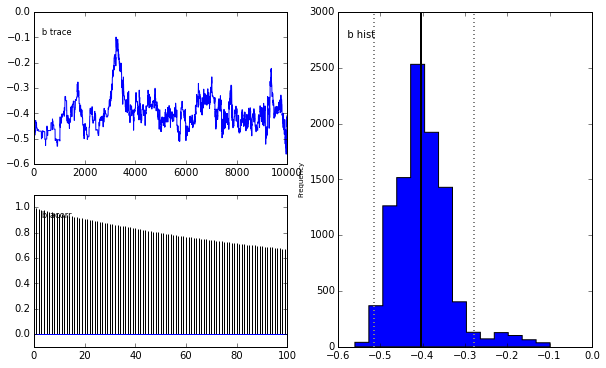## Example: Coal mining disasters¶

Recall the earlier example of estimating a changepoint in the time series of UK coal mining disasters.

In :
import matplotlib.pyplot as plt
from pymc.examples.disaster_model import disasters_array

plt.figure(figsize=(12.5, 3.5))
n_count_data = len(disasters_array)
plt.bar(np.arange(1851, 1962), disasters_array, color="#348ABD")
plt.xlabel("Year")
plt.ylabel("Disasters")
plt.title("UK coal mining disasters, 1851-1962")
plt.xlim(1851, 1962);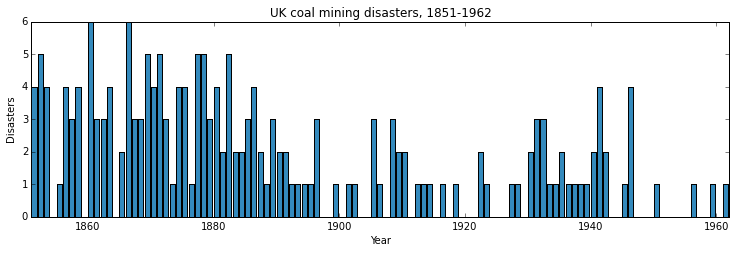We represent our conceptual model formally as a statistical model:

$$\begin{array}{ccc} (y_t | \tau, \lambda_1, \lambda_2) \sim\text{Poisson}\left(r_t\right), & r_t=\left\{ \begin{array}{lll} \lambda_1 &\text{if}& t< \tau\\ \lambda_2 &\text{if}& t\ge \tau \end{array}\right.,&t\in[t_l,t_h]\\ \tau \sim \text{DiscreteUniform}(t_l, t_h)\\ \lambda_1\sim \text{Exponential}(a)\\ \lambda_2\sim \text{Exponential}(b) \end{array}$$

Because we have defined $y$ by its dependence on $\tau$, $\lambda_1$ and $\lambda_2$, the latter three are known as the parents of $y$ and $D$ is called their child. Similarly, the parents of $\tau$ are $t_l$ and $t_h$, and $\tau$ is the child of $t_l$ and $t_h$.

## PyMC Variables¶

At the model-specification stage (before the data are observed), $y$, $\tau$, $\lambda_1$, and $\lambda_2$ are all random variables. Recall from the discussion of subjective probability that the Bayesian interpretation of probability is epistemic, meaning random variable $x$'s probability distribution $p(x)$ represents our knowledge and uncertainty about $x$'s value, rather than some random generative process. Candidate values of $x$ for which $p(x)$ is high are relatively more probable, given what we know. Random variables are represented in PyMC by the classes Stochastic and Deterministic.

The only Deterministic in the model is $r$. If we knew the values of $r$'s parents, we could compute the value of $r$ exactly. A Deterministic like $r$ is defined by a mathematical function that returns its value given values for its parents. Deterministic variables are sometimes called the systemic part of the model. The nomenclature is a bit confusing, because these objects usually represent random variables; since the parents of $r$ are random, $r$ is random also.

On the other hand, even if the values of the parents of variables switchpoint, disasters (before observing the data), early_mean or late_mean were known, we would still be uncertain of their values. These variables are characterized by probability distributions that express how plausible their candidate values are, given values for their parents. The Stochastic class represents these variables.

First, we represent the unknown switchpoint as a discrete uniform random variable:

In :
from pymc import DiscreteUniform, Exponential, Poisson, deterministic

switchpoint = DiscreteUniform('switchpoint', lower=0, upper=110)

In :
switchpoint

Out:
<pymc.distributions.DiscreteUniform 'switchpoint' at 0x102452850>

DiscreteUniform is a subclass of Stochastic that represents uniformly-distributed discrete variables. Use of this distribution suggests that we have no preference a priori regarding the location of the switchpoint; all values are equally likely.

Now we create the exponentially-distributed variables early_mean and late_mean for the early and late Poisson rates, respectively:

In :
early_mean = Exponential('early_mean', beta=1., value=3)
late_mean = Exponential('late_mean', beta=1., value=1)


Next, we define the variable rate, which selects the early rate early_mean for times before switchpoint and the late rate late_mean for times after switchpoint. We create rate using the deterministic decorator, which converts the ordinary Python function rate into a Deterministic object.

In :
@deterministic
def rate(s=switchpoint, e=early_mean, l=late_mean):
# Create a vector of Poisson means
out = np.empty(len(disasters_array))
out[:s] = e
out[s:] = l
return out


The last step is to define the number of disasters disasters. This is a stochastic variable but unlike switchpoint, early_mean and late_mean we have observed its value. To express this, we set the argument observed to True (it is set to False by default). This tells PyMC that this object's value should not be changed:

In :
disasters = Poisson('disasters', mu=rate, value=disasters_array,
observed=True)


### Why are data and unknown variables represented by the same object?¶

Since its represented by a Stochastic object, disasters is defined by its dependence on its parent rate even though its value is fixed. This isn't just a quirk of PyMC's syntax; Bayesian hierarchical notation itself makes no distinction between random variables and data. The reason is simple: to use Bayes' theorem to compute the posterior, we require the likelihood. Even though disasters's value is known and fixed, we need to formally assign it a probability distribution as if it were a random variable.

Remember, the likelihood and the probability function are essentially the same, except that the former is regarded as a function of the parameters and the latter as a function of the data.

## Parents and children¶

We have above created a PyMC probability model, which is simply a linked collection of variables. To see the nature of the links, we can examine any node's parents attribute:

In :
switchpoint.parents

Out:
{'lower': 0, 'upper': 110}

The parents dictionary shows us the distributional parameters of switchpoint, which are constants. Now let's examine the parents of disasters and rate:

In :
disasters.parents

Out:
{'mu': <pymc.PyMCObjects.Deterministic 'rate' at 0x107dd3cd0>}
In :
rate.parents

Out:
{'e': <pymc.distributions.Exponential 'early_mean' at 0x107dd3810>,
'l': <pymc.distributions.Exponential 'late_mean' at 0x107dd38d0>,
's': <pymc.distributions.DiscreteUniform 'switchpoint' at 0x102452850>}

We are using rate as a distributional parameter of disasters (i.e. rate is disasters's parent). disasters internally labels rate as mu, meaning rate plays the role of the rate parameter in disasters's Poisson distribution. Now examine rate's children attribute:

In :
rate.children

Out:
{<pymc.distributions.Poisson 'disasters' at 0x107dd3690>}

Because disasters considers rate its parent, rate considers disasters its child. Unlike parents, children is a set (an unordered collection of objects); variables do not associate their children with any particular distributional role, so an index is not required. Try examining the parents and children attributes of the other parameters in the model.

The following directed acyclic graph is a visualization of the parent-child relationships in the model. Unobserved stochastic variables switchpoint, early_mean and late_mean are open ellipses, observed stochastic variable disasters is a filled ellipse and deterministic variable rate is a triangle. Arrows point from parent to child and display the label that the child assigns to the parent.

In :
from pymc import graph, MCMC
graph.dag(MCMC([switchpoint, rate, early_mean, late_mean, disasters]))

Out:
<pydot.Dot at 0x107dd3b90>
In :
!dot MCMC.dot -Tpng -o images/dag.png
from IPython.core.display import Image
Image('images/dag.png')

Out: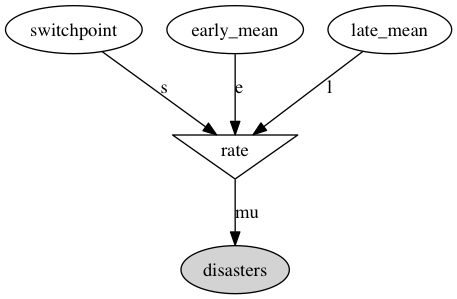As the examples above have shown, PyMC objects need to have a name assigned, such as switchpoint, early_mean or late_mean. These names are used for storage and post-processing:

• as keys in on-disk databases,
• as node labels in model graphs,
• as axis labels in plots of traces,
• as table labels in summary statistics.

A model instantiated with variables having identical names raises an error to avoid name conflicts in the database storing the traces. In general however, PyMC uses references to the objects themselves, not their names, to identify variables.

## Variables' values and log-probabilities¶

All PyMC variables have an attribute called value that stores the current value of that variable. Try examining disasters's value, and you'll see the dataset we provided for it:

In :
disasters.value

Out:
array([4, 5, 4, 0, 1, 4, 3, 4, 0, 6, 3, 3, 4, 0, 2, 6, 3, 3, 5, 4, 5, 3, 1,
4, 4, 1, 5, 5, 3, 4, 2, 5, 2, 2, 3, 4, 2, 1, 3, 2, 2, 1, 1, 1, 1, 3,
0, 0, 1, 0, 1, 1, 0, 0, 3, 1, 0, 3, 2, 2, 0, 1, 1, 1, 0, 1, 0, 1, 0,
0, 0, 2, 1, 0, 0, 0, 1, 1, 0, 2, 3, 3, 1, 1, 2, 1, 1, 1, 1, 2, 4, 2,
0, 0, 1, 4, 0, 0, 0, 1, 0, 0, 0, 0, 0, 1, 0, 0, 1, 0, 1])

If you check the values of early_mean, switchpoint and late_mean, you'll see random initial values generated by PyMC:

In :
switchpoint.value

Out:
array(60)
In :
early_mean.value

Out:
array(3.0)
In :
late_mean.value

Out:
array(1.0)

Of course, since these are Stochastic elements, your values will be different than these. If you check rate's value, you'll see an array whose first switchpoint elements are early_mean, and whose remaining elements are late_mean:

In :
rate.value

Out:
array([ 3.,  3.,  3.,  3.,  3.,  3.,  3.,  3.,  3.,  3.,  3.,  3.,  3.,
3.,  3.,  3.,  3.,  3.,  3.,  3.,  3.,  3.,  3.,  3.,  3.,  3.,
3.,  3.,  3.,  3.,  3.,  3.,  3.,  3.,  3.,  3.,  3.,  3.,  3.,
3.,  3.,  3.,  3.,  3.,  3.,  3.,  3.,  3.,  3.,  3.,  3.,  3.,
3.,  3.,  3.,  3.,  3.,  3.,  3.,  3.,  1.,  1.,  1.,  1.,  1.,
1.,  1.,  1.,  1.,  1.,  1.,  1.,  1.,  1.,  1.,  1.,  1.,  1.,
1.,  1.,  1.,  1.,  1.,  1.,  1.,  1.,  1.,  1.,  1.,  1.,  1.,
1.,  1.,  1.,  1.,  1.,  1.,  1.,  1.,  1.,  1.,  1.,  1.,  1.,
1.,  1.,  1.,  1.,  1.,  1.,  1.])

To compute its value, rate calls the function we used to create it, passing in the values of its parents.

Stochastic objects can evaluate their probability mass or density functions at their current values given the values of their parents. The logarithm of a stochastic object's probability mass or density can be accessed via the logp attribute. For vector-valued variables like disasters, the logp attribute returns the sum of the logarithms of the joint probability or density of all elements of the value.

Try examining switchpoint's and disasters's log-probabilities and early_mean 's and late_mean's log-densities:

In :
switchpoint.logp

Out:
-4.709530201312334
In :
late_mean.value = 5

In :
disasters.logp

Out:
-318.0083429843733
In :
early_mean.logp

Out:
-3.0
In :
late_mean.logp

Out:
-5.0

Stochastic objects need to call an internal function to compute their logp attributes, as rate needed to call an internal function to compute its value. Just as we created rate by decorating a function that computes its value, it's possible to create custom Stochastic objects by decorating functions that compute their log-probabilities or densities (see chapter :ref:chap_modelbuilding). Users are thus not limited to the set of of statistical distributions provided by PyMC.

### Using Variables as parents of other Variables¶

Let's take a closer look at our definition of rate:

@deterministic(plot=False)
def rate(s=switchpoint, e=early_mean, l=late_mean):
''' Concatenate Poisson means '''
out = empty(len(disasters_array))
out[:s] = e
out[s:] = l
return out



The arguments switchpoint, early_mean and late_mean are Stochastic objects, not numbers. If that is so, why aren't errors raised when we attempt to slice array out using a Stochastic object?

Whenever a variable is used as a parent for a child variable, PyMC replaces it with its value attribute when the child's value or log-probability is computed. When rate's value is recomputed, s.value is passed to the function as argument switchpoint. To see the values of the parents of rate all together, look at rate.parents.value.

## Fitting the model with MCMC¶

PyMC provides several objects that fit probability models (linked collections of variables) like ours. The primary such object, MCMC, fits models with a Markov chain Monte Carlo algorithm:

In :
from pymc.examples import disaster_model
M = MCMC(disaster_model)


In this case M will expose variables switchpoint, early_mean, late_mean and disasters as attributes; that is, M.switchpoint will be the same object as disaster_model.switchpoint.

To run the sampler, call the MCMC object's sample() (or isample(), for interactive sampling outside of the IPython notebook) method with arguments for the number of iterations, burn-in length, and thinning interval (if desired):

In :
M.sample(iter=10000, burn=1000)

 [-----------------100%-----------------] 10000 of 10000 complete in 1.6 sec

### Accessing the samples¶

The output of the MCMC algorithm is a trace, the sequence of retained samples for each variable in the model. These traces can be accessed using the trace(name, chain=-1) method. For example:

In :
M.trace('switchpoint')[1000:]

Out:
array([39, 39, 39, ..., 41, 41, 41])

The trace slice [start:stop:step] works just like the NumPy array slice. By default, the returned trace array contains the samples from the last call to sample, that is, chain=-1, but the trace from previous sampling runs can be retrieved by specifying the correspondent chain index. To return the trace from all chains, simply use chain=None.

A node's chain can be accessed directly using its trace attribute:

In :
M.early_mean.trace()

Out:
array([ 2.60698391,  2.60698391,  2.60428494, ...,  2.79480505,
2.72259973,  2.69514232])

### Sampling output¶

You can examine the marginal posterior of any variable by plotting a histogram of its trace:

In :
plt.hist(M.trace('late_mean')[:])

Out:
(array([   20.,   151.,   706.,  1645.,  2300.,  2042.,  1255.,   623.,
215.,    43.]),
array([ 0.55752905,  0.63378676,  0.71004447,  0.78630219,  0.8625599 ,
0.93881762,  1.01507533,  1.09133304,  1.16759076,  1.24384847,
1.32010619]),
<a list of 10 Patch objects>)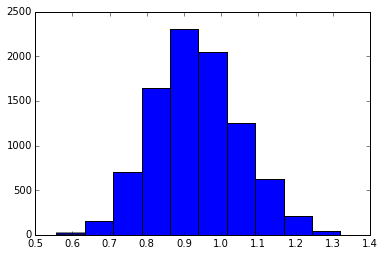PyMC has its own plotting functionality, via the optional matplotlib module as noted in the installation notes. The Matplot module includes a plot function that takes the model (or a single parameter) as an argument:

In :
from pymc.Matplot import plot
plot(M)

Plotting switchpoint
Plotting late_mean
Plotting early_mean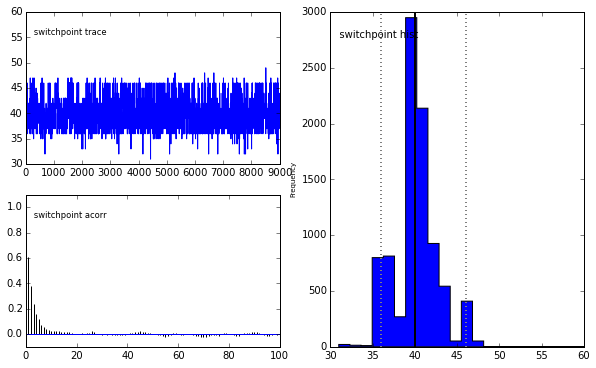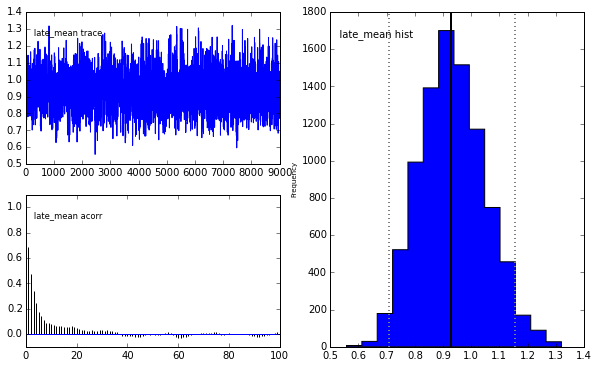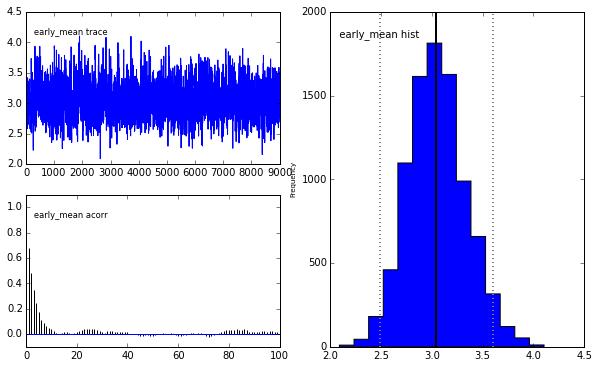The upper left-hand pane of each figure shows the temporal series of the samples from each parameter, while below is an autocorrelation plot of the samples. The right-hand pane shows a histogram of the trace. The trace is useful for evaluating and diagnosing the algorithm's performance, while the histogram is useful for visualizing the posterior.

An alternative visualization of posterior quantities is to use the summary_plot function to create forest plots of stochastic or determinsitic nodes. This will display the posterior median, interquartile range, and posterior credible interval (defaults to 95%) in a layout that makes it easy to compare multiple nodes.

In :
Matplot.summary_plot([M.early_mean, M.late_mean])

Could not calculate Gelman-Rubin statistics. Requires multiple chains of equal length.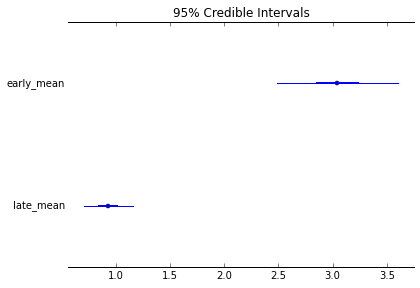For a non-graphical summary of the posterior, simply call the summary method.

In :
M.early_mean.stats()

Out:
{'95% HPD interval': array([ 2.49124983,  3.60405374]),
'mc error': 0.00756500947969721,
'mean': 3.0527065227383434,
'n': 9000,
'quantiles': {2.5: 2.5172050574749441,
25: 2.8605823290604975,
50: 3.0380496362489353,
75: 3.2300775101774053,
97.5: 3.6363693972833957},
'standard deviation': 0.28702076399829468}

### Imputation of Missing Data¶

As with most textbook examples, the models we have examined so far assume that the associated data are complete. That is, there are no missing values corresponding to any observations in the dataset. However, many real-world datasets have missing observations, usually due to some logistical problem during the data collection process. The easiest way of dealing with observations that contain missing values is simply to exclude them from the analysis; this is called a complete case analysis. However, this results in loss of information if an excluded observation contains valid values for other quantities, and can bias results. An alternative is to impute the missing values, based on information in the rest of the model.

For example, consider a survey dataset for some wildlife species:

Count   Site   Observer   Temperature
------- ------ ---------- -------------
15      1      1          15
10      1      2          NA
6       1      1          11



Each row contains the number of individuals seen during the survey, along with three covariates: the site on which the survey was conducted, the observer that collected the data, and the temperature during the survey. If we are interested in modelling, say, population size as a function of the count and the associated covariates, it is difficult to accommodate the second observation because the temperature is missing (perhaps the thermometer was broken that day). Ignoring this observation will allow us to fit the model, but it wastes information that is contained in the other covariates.

In a Bayesian modelling framework, missing data are accommodated simply by treating them as unknown model parameters. Values for the missing data $\tilde{y}$ are estimated naturally, using the posterior predictive distribution:

$$p(\tilde{y}|y) = \int p(\tilde{y}|\theta) f(\theta|y) d\theta$$

This describes additional data $\tilde{y}$, which may either be considered unobserved data or potential future observations. We can use the posterior predictive distribution to model the likely values of missing data, assuming values are missing completely at random.

Consider the coal mining disasters data introduced previously. Assume that two years of data are missing from the time series; we indicate this in the data array by the use of an arbitrary placeholder value, None:

In :
x = np.array([ 4, 5, 4, 0, 1, 4, 3, 4, 0, 6, 3, 3, 4, 0, 2, 6,
3, 3, 5, 4, 5, 3, 1, 4, 4, 1, 5, 5, 3, 4, 2, 5,
2, 2, 3, 4, 2, 1, 3, None, 2, 1, 1, 1, 1, 3, 0, 0,
1, 0, 1, 1, 0, 0, 3, 1, 0, 3, 2, 2, 0, 1, 1, 1,
0, 1, 0, 1, 0, 0, 0, 2, 1, 0, 0, 0, 1, 1, 0, 2,
3, 3, 1, None, 2, 1, 1, 1, 1, 2, 4, 2, 0, 0, 1, 4,
0, 0, 0, 1, 0, 0, 0, 0, 0, 1, 0, 0, 1, 0, 1])


To estimate these values in PyMC, we generate a masked array. These are specialised NumPy arrays that contain a matching True or False value for each element to indicate if that value should be excluded from any computation. Masked arrays can be generated using NumPy's ma.masked_equal function:

In :
masked_values = np.ma.masked_values(x, value=None)

Out:
masked_array(data = [4 5 4 0 1 4 3 4 0 6 3 3 4 0 2 6 3 3 5 4 5 3 1 4 4 1 5 5 3 4 2 5 2 2 3 4 2
1 3 -- 2 1 1 1 1 3 0 0 1 0 1 1 0 0 3 1 0 3 2 2 0 1 1 1 0 1 0 1 0 0 0 2 1 0
0 0 1 1 0 2 3 3 1 -- 2 1 1 1 1 2 4 2 0 0 1 4 0 0 0 1 0 0 0 0 0 1 0 0 1 0 1],
mask = [False False False False False False False False False False False False
False False False False False False False False False False False False
False False False False False False False False False False False False
False False False  True False False False False False False False False
False False False False False False False False False False False False
False False False False False False False False False False False False
False False False False False False False False False False False  True
False False False False False False False False False False False False
False False False False False False False False False False False False
False False False],
fill_value = ?)

This masked array, in turn, can then be passed to one of PyMC's data stochastic variables, which recognizes the masked array and replaces the missing values with Stochastic variables of the desired type. For the coal mining disasters problem, recall that disaster events were modeled as Poisson variates:

In :
disasters = Poisson('disasters', mu=rate,


Here rate is an array of means for each year of data, allocated according to the location of the switchpoint. Each element in disasters is a Poisson Stochastic, irrespective of whether the observation was missing or not. The difference is that actual observations are data Stochastics (observed=True), while the missing values are non-data Stochastics. The latter are considered unknown, rather than fixed, and therefore estimated by the MCMC algorithm, just as unknown model parameters.

The entire model looks very similar to the original model:

In :
def missing_data_model():

# Switchpoint
switch = DiscreteUniform('switch', lower=0, upper=110)
# Early mean
early_mean = Exponential('early_mean', beta=1)
# Late mean
late_mean = Exponential('late_mean', beta=1)

@deterministic(plot=False)
def rate(s=switch, e=early_mean, l=late_mean):
"""Allocate appropriate mean to time series"""
out = np.empty(len(disasters_array))
# Early mean prior to switchpoint
out[:s] = e
# Late mean following switchpoint
out[s:] = l
return out

# Pass masked array to data stochastic, and it does the right thing
disasters = Poisson('disasters', mu=rate, value=masked_values, observed=True)

return locals()


Here, we have used the masked_values function, rather than masked_equal; The result is the same.

In :
M.early_mean.summary()

early_mean:

Mean             SD               MC Error        95% HPD interval
------------------------------------------------------------------
3.053            0.287            0.008            [ 2.491  3.604]

Posterior quantiles:

2.5             25              50              75             97.5
|---------------|===============|===============|---------------|
2.517            2.861           3.038          3.23          3.636


In :
M_missing = MCMC(missing_data_model())
M_missing.sample(5000)

 [-----------------100%-----------------] 5000 of 5000 complete in 1.3 sec
In :
Matplot.plot(M_missing.disasters)

Plotting disasters_0
Plotting disasters_1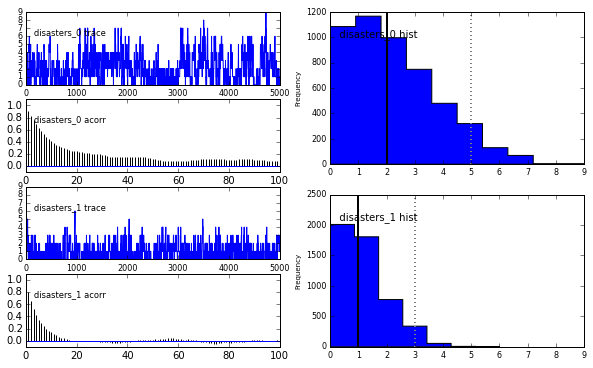## Fine-tuning the MCMC algorithm¶

MCMC objects handle individual variables via step methods, which determine how parameters are updated at each step of the MCMC algorithm. By default, step methods are automatically assigned to variables by PyMC. To see which step methods $M$ is using, look at its step_method_dict attribute with respect to each parameter:

In :
M.step_method_dict

Out:
{<pymc.distributions.Exponential 'late_mean' at 0x107a034d0>: [<pymc.StepMethods.Metropolis at 0x107a2f710>],
<pymc.distributions.Exponential 'early_mean' at 0x107a035d0>: [<pymc.StepMethods.Metropolis at 0x107a2f750>],
<pymc.distributions.DiscreteUniform 'switchpoint' at 0x107a13810>: [<pymc.StepMethods.DiscreteMetropolis at 0x107a2fc10>]}

The value of step_method_dict corresponding to a particular variable is a list of the step methods $M$ is using to handle that variable.

You can force $M$ to use a particular step method by calling M.use_step_method before telling it to sample. The following call will cause $M$ to handle late_mean with a Slicer (slice sampling) step method, and assigns an initial slice width w=0.5:

In :
from pymc import Slicer
M.use_step_method(Slicer, disaster_model.late_mean, w=0.5)


Another step method class, AdaptiveMetropolis, is better at handling highly-correlated variables. If your model mixes poorly, using AdaptiveMetropolis is a sensible first thing to try.

In :
from IPython.core.display import HTML
def css_styling():